WEBINAR
Understanding Eccentricity Effects on Stator Tooth Forces for eNVH Analysis
Thursday, June 8, 2023
Time
SESSION 1
SESSION 2
CEST (GMT +2)
03:00 PM
08:00 PM
EDT (GMT -4)
09:00 AM
02:00 PM
HOME / Applications / PERMANENT MAGNETS GEAR SYSTEM: 2D AND 3D ELECTROMAGNETIC SIMULATION INSIDE SOLIDWORKS

# PERMANENT MAGNETS GEAR SYSTEM: 2D AND 3D ELECTROMAGNETIC SIMULATION INSIDE SOLIDWORKS

## Magnetic Gear

Magnetic gears (MG) which achieve contactless power transmission are increasingly attracting the interest of industry. Traditional mechanical gears suffer from several weaknesses:  friction losses, lubrication issues, heat, vibration and noise. Magnetic gears are therefore developed to overcome the issues accompanying the use of traditional mechanical ones.

They are characterized by a frictionless energy transmission which saves lubrication and maintenance costs and minimizes heat issues. Moreover, MG are recognized for their role in reducing noise and vibration transmission and many industrial fields such as, robotics, automotive, oil and gas, marine propulsion have benefited from the high efficiency and reliability of magnetic gears.
Engineers have been working on designing and developing highly efficient and high-performance magnetic gears. Different topologies are proposed in Figure 1.

CAD design and numerical simulation helps to design and analyze magnetic gear systems to improve their efficiency. Thus, finite element method (FEM) is used thanks to its reliability to compute magnetic results, i.e., magnetic flux density, magnetic field intensity, force, torque, etc.
In addition to 3D, 2D FEM simulation is used in problems representing either planar or rotational symmetry.

In this article, a coaxial magnetic gear system proposed in Figure  is studied. Since the model geometry has planar symmetry, both 2D and 3D simulations are performed using EMWorks2D and EMS, successively. 2D Torque results will be compared to published data . A comparison of 2D and 3D results will be established at the end of this article.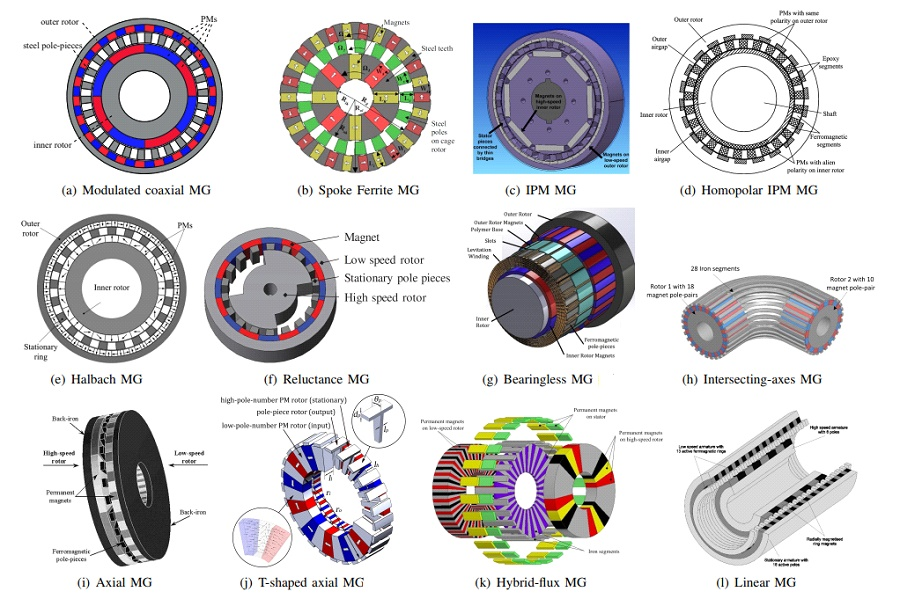Figure 1 - Schemes of various conventional magnetic gears

## 2D FEM simulation of coaxial magnetic gear 

A coaxial magnetic gear model is presented in . The proposed MG is made of 4 pole-pairs in the inner rotor, 22 pole-pairs in the outer rotor, and 26 modulating pole-pieces. So, the model has a gear ratio of.
The rotation speed of the inner rotor is higher than the outer rotor. The low speed rotor develops higher torque than the high-speed rotor.

The magnetic flux in the air gap region and the developed torque is evaluated using EMWorks2D.
Both eddy and end effects are neglected in this analysis.  Figure 2 shows the structure and the dimensions of the magnetic gear model.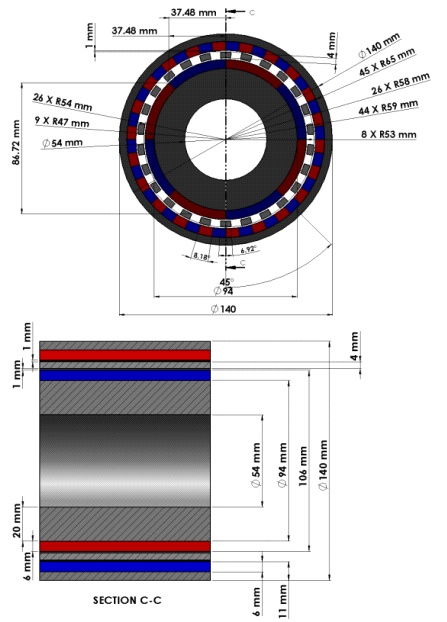Figure 2 - MG geometry 

The model is created inside SOLIDWORKS and displayed in Figure 3. The inner rotor is rotating with the speed ofrpm while the speed of the outer rotor isrpm. The rotor cores and the modulator are made of steel 1008 characterized by the BH curve demonstrated in Figure 4.  NdFeB 35 permanent magnets are used and their properties are presented in table 1.

A parametric Magnetostatic simulation is defined using EMWorks2D where the angles of both inner and outer rotors are varied. Figures 3a) and 3b) show the mesh in the model and the air gap. Triangles mesh elements are automatically generated with EMWorks2D. Moreover, it allows to define specific mesh elements size in predefined surface regions or edges.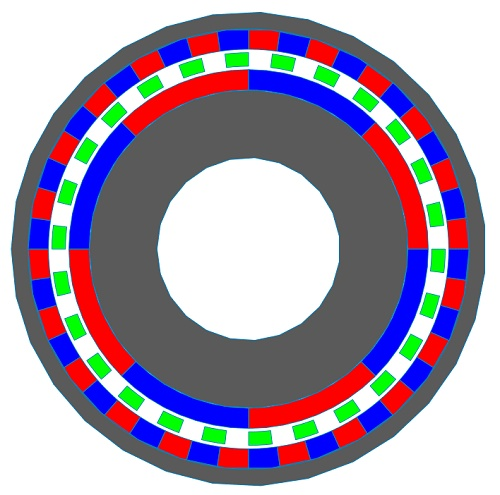Figure 3 - 2D model of the simulated magnetic gear system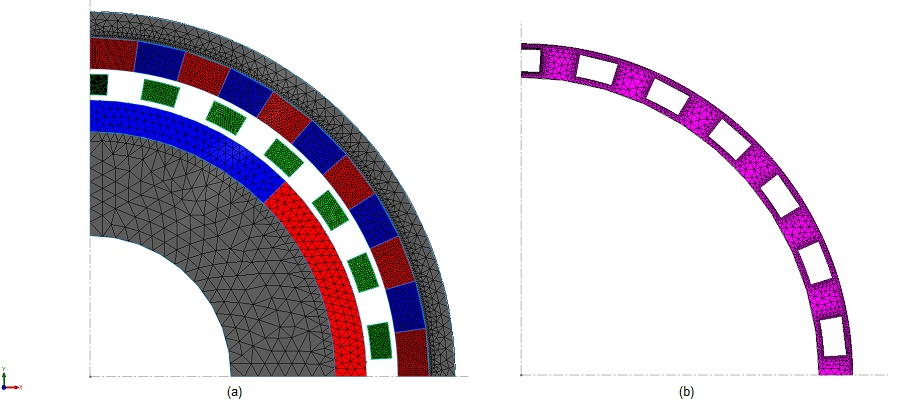Figure 4 - Generated mesh a) in the model, b) in the air gap region

During the simulation, the angles of the inner and outer rotor are linked by the following equation:. Fringe, vector and line plots of the magnetic flux density are exhibited in Figures 4a), 4b) and 4c) (and), respectively. The magnetic field plots are characterized by high flux spots in the ferromagnetic pieces.

Both field lines and vector plots can help  to identify and verify the flux directions. Figures 5 and 6 illustrate fringe plot of the magnetic field intensity and contour lines plot of the magnetic vector potential.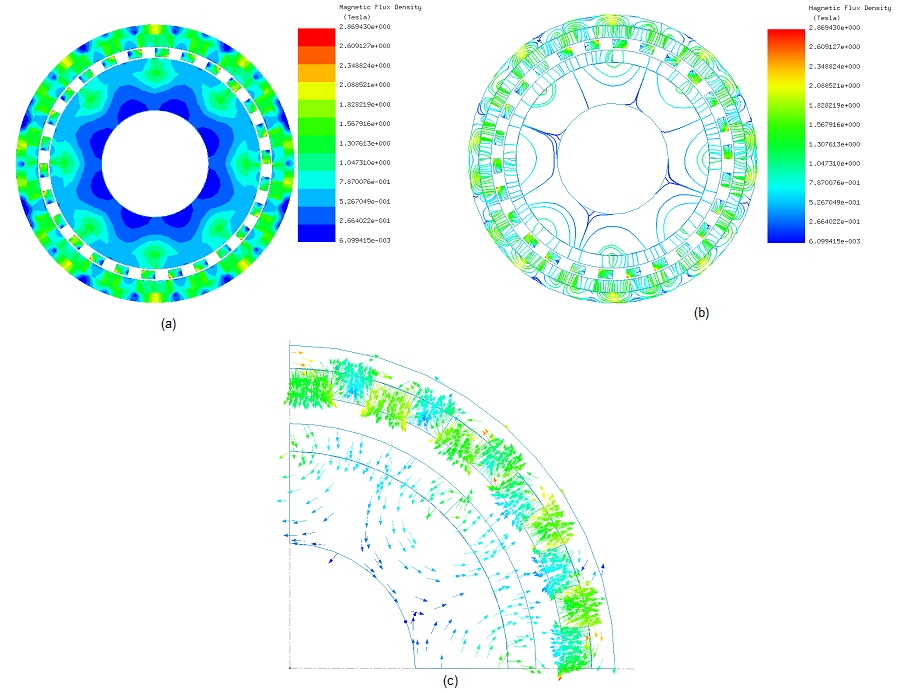Figure 5 - Magnetic flux density, a) fringe, b) line, c) vector plots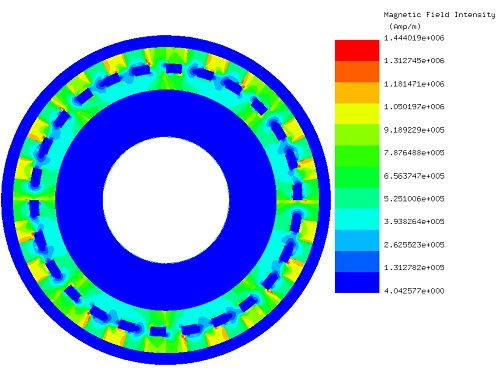Figure 6 - Fringe plot of the magnetic field intensity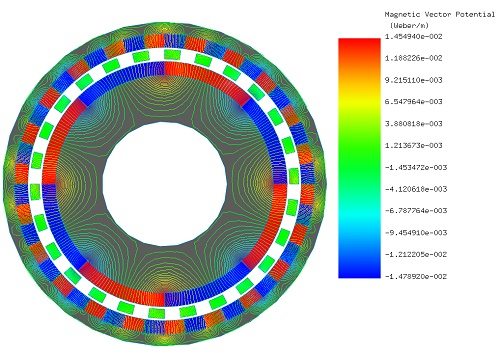Figure 7 - Contour lines plot of the magnetic vector potential

Figures 7a) and 7b) depict the generated torque results respectively in the outer and inner rotors. As mentioned above, the outer rotor with lower speed develops higher torque than the inner rotor. Due to the high poles-pairs in the outer rotor less ripples are obtained in the torque curve in figure 7a) compared to those in Figure 7b) .

At the initial angular position, the outer rotor torque is about 1737 N.m, while it is around 315 N.m in the inner rotor. Thus, they verify the gear ratio relation between the inner and outer rotors.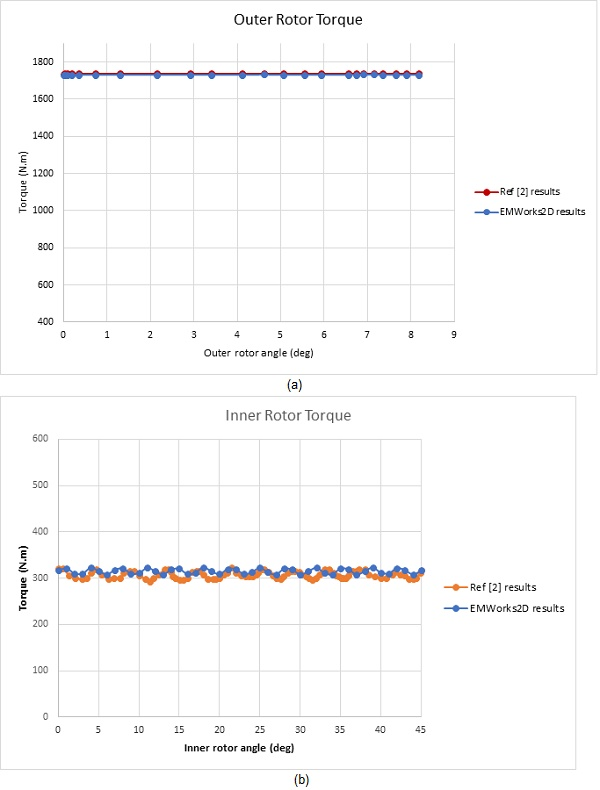Figure 8 - Torque results, a) outer rotor, b) inner rotor

## 3D FEM simulation of the coaxial magnetic gear

The same proposed magnetic gear model studied with EMWorks2D in the previous section is now analyzed using the 3D electromagnetic simulation software EMS, keeping the same model dimensions. Since the model represents an axial symmetry, it is recommended to run only a portion of it. In this article, a 20 mm magnetic gear is simulated instead of the 300 mm length of the original model which subsequently reduces the computational time. Figure 8 shows the 3D model of the studied geometry built inside SOLIDWORKS.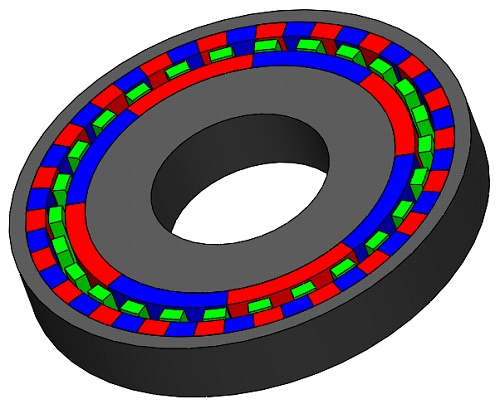Figure 9 - 3D model of magnetic gear device

The meshed model is presented in Figure 8. It is meshed with tetrahedral mesh elements recognized for their accurate solution. Figures 9a) and 9b) contain the magnetic flux density fringe and vector plots, consecutively.

The Iso surfaces of the magnetic flux density in Figure 10 are plotted in the inner rotor, while Figure 11 illustrates a plot of the magnetic field along a line between two poles of the outer rotor. The curve has a symmetric shape regarding the model center. So, only half of the curve is interpreted. The field is maximum at the extremity (at the magnet surface of the outer rotor), then it drops down when passing through the air gap zone to increase until reaching two peaks in the inner rotor magnet. Eventually, it decreases again until becoming close to zero in the shaft (it is assumed as air in the current simulation).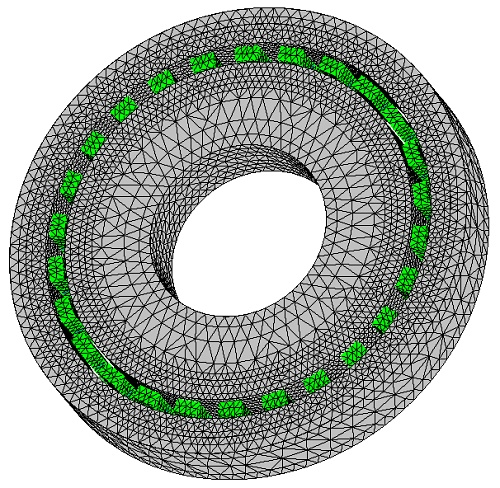Figure 10 - 3D meshed model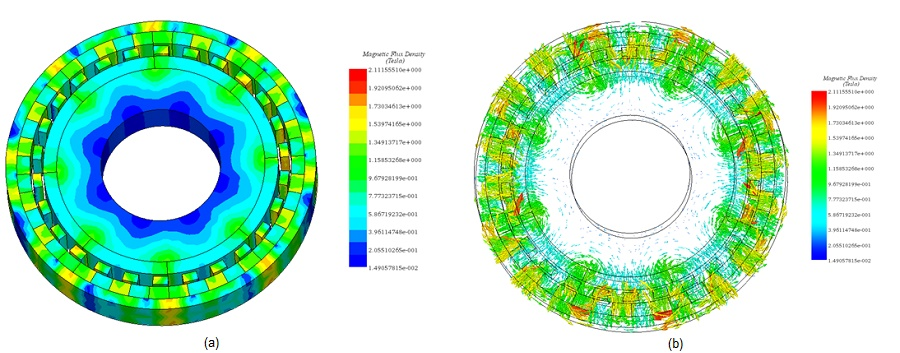Figure 11 - Magnetic flux density, a) fringe plot, b) vector plot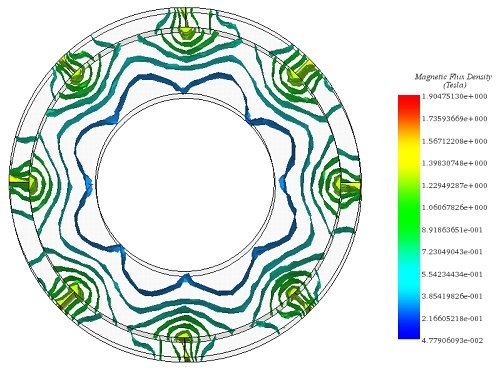Figure 12 - Iso surface plot of the magnetic flux density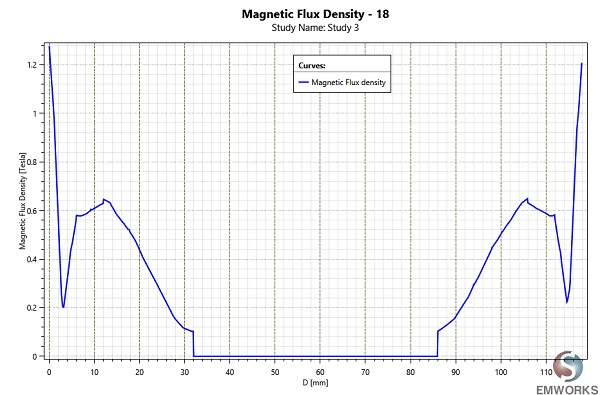Figure 13 - Magnetic flux density along line

Figures 12a) and 12b) reveal the torque results in both the outer and inner rotors. Analogous to 2D results computed by EMWorks2D, the generated torque in the low-speed torque is around 1730 N.m while it  reached  310 N.m in the high-speed rotor.

As it can be concluded from Figures 7 and 12, 2D simulation results corroborate those computed with 3D FEM software EMS. Figure 13 contains an animation plot of the magnetic flux density versus the angles of both inner and outer rotors. It shows that inner and outer rotors are spinning in opposite direction.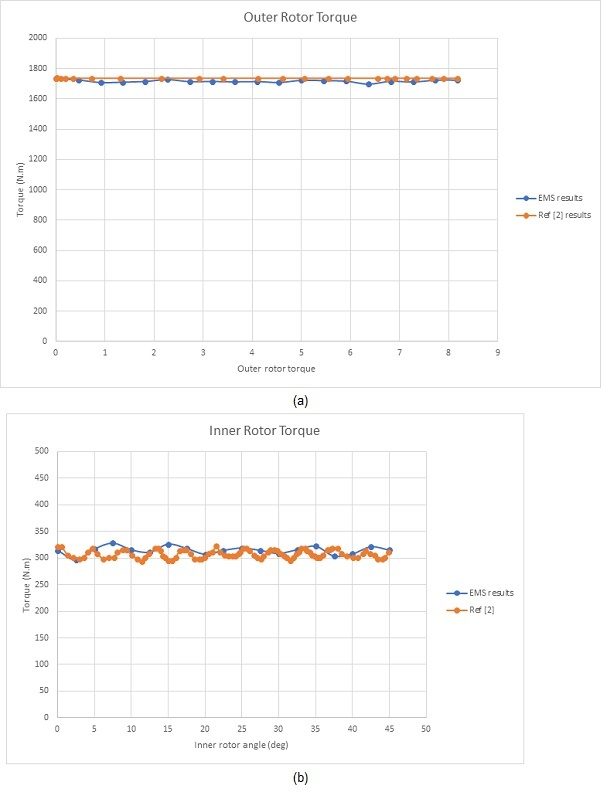Figure 14 - Torque results, a) outer rotor, b) inner rotor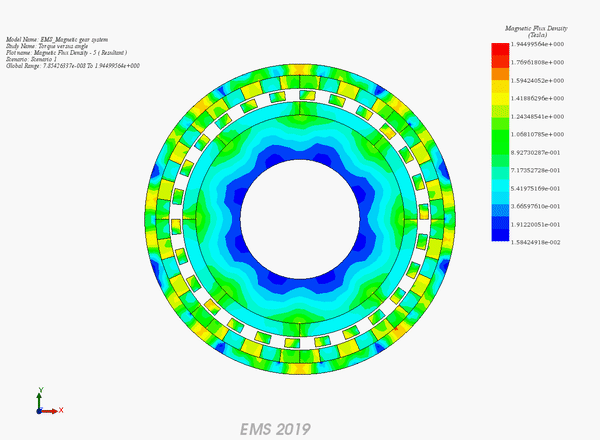Figure 15 - Animation plot of the magnetic flux density versus angle

## Conclusion

The use of magnetic gear systems is witnessing a substantial spread throughout various fields and applications. Users prefer MG for their numerous advantages compared to traditional mechanical gears. Furthermore, numerical simulation contributes to the design and creation of innovative, robust, and efficient magnetic gears. Both 2D and 3D FE analyses can be used in earlier stages to predict magnetic results like magnetic flux density, force, torque, etc. Both EMWorks2D and EMS are used in this application note to compute magnetic flux density and torque results for different components as well as different configurations of the studied magnetic gear. In the first section, 2D FE simulation were established to evaluate the torque of both inner and outer rotors versus their positions. The 3D simulation performed on the model  came to emphasizes the accuracy of the 2D results which logically   justifies   the use of the approximative 2D solution.

#### References

: Yawei Wang, Mattia Filippini, Nicola Bianchi, Piergiorgio Alotto. A Review on Magnetic Gears: Topologies, Computational Models and Design Aspects. Published in: 2018 XIII International Conference on Electrical Machines (ICEM), IEEE
: Valentin Mateev, Iliana Marinova and Miglenna Todorova Department of Electrical Apparatus Technical University of Sofia, Bulgaria. Eddy current losses in permanent magnets of a coaxial magnetic gear. Published in: 2018 20th International Symposium on Electrical Apparatus and Technologies (SIELA), IEEE